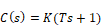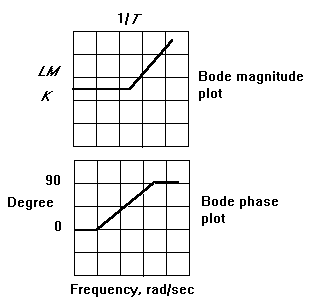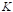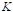### Proportional - derivative (PD) compensator

Transfer functionBode plotsThe characteristics of the Bode plots are summarized in the following PD table:

 Frequency LM Phase <<<1/T0 1/T45 >>>1/T ∞ 90

To design a PD compensator

1.    Using the PD table, determine the frequency (ω∗) where phase is to be added, and determine the amount of phase to be added.

2.    Select the PD zero at the frequency that produces the desired phase (and interpolate where necessary).

3.    Implement the compensator and adjust the K gain for a satisfactory transient response, beginning with K = 1.

Although the PD compensator provides phase lead at frequencies above 1/T, it also adds more and more amplification as frequency increases. When noise is present, as it usually is, this is an undesirable property because the PD compensator amplifies the noise to a large degree.